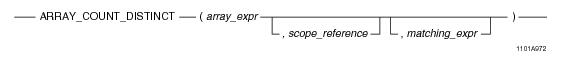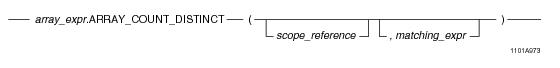# 15.00 - ARRAY_COUNT_DISTINCT - Teradata Database

## Teradata Database SQL Functions, Operators, Expressions, and Predicates

Product
Release Number
15.00
Content Type
Programming Reference
Publication ID
B035-1145-015K
Language
English (United States)

## ARRAY_COUNT_DISTINCT

### Purpose

Returns the number of distinct elements in array_expr, optionally matching a specific input value.

### Syntax

System function syntax:Method-style syntax:where:

 Syntax element… Specifies… array_expr one of the following: A reference to an ARRAY column An ARRAY constructor expression. See “ARRAY Constructor Expression” on page 195. A UDF expression A UDM expression The element type of the array must be a numeric or character data type. scope_reference an optional ARRAY scope reference. See “ARRAY Scope Reference” on page 198. matching_expr an optional expression to be matched by one or more elements in the array.

### ANSI Compliance

This is a Teradata extension to the ANSI SQL:2011 standard.

### Usage Notes

ARRAY_COUNT_DISTINCT takes an array expression as an argument and returns a value that represents one of two possible outputs:

• If you specify the optional argument matching_expr, then the function returns the number of elements in array_expr whose values are equal to matching_expr. If matching_expr is NULL, the function returns the number of elements with NULLs.
• If you do not specify matching_expr, then the function returns the number of distinct elements in array_expr. If a NULL element is encountered in the array argument, it is ignored and not considered when doing the calculation.
• If you specify a scope reference, the function is applied only to the elements within the limits of the given scope. You can use ARRAY_COUNT_DISTINCT with arrays of numeric as well as character element types.

If the array argument contains any elements that are in an uninitialized state, an error is returned. Use a scope reference to avoid referencing a range of the array with uninitialized elements, or set any uninitialized elements to NULL. You can do this with the OEXTEND method. See “OEXTEND” on page 272.

If array_expr is NULL, the function returns NULL.

### Result Type

The result type is an INTEGER.

### Example

Consider the following 1-D ARRAY data type and table.

`CREATE TYPE item_price AS DECIMAL(7,2) ARRAY;`
` `
`CREATE TABLE inventory (itemkind INTEGER,`
`                        regular_price item_price,`
`                        sale_price item_price);`

In the following query, ARRAY_COUNT_DISTINCT returns the number of elements whose value is equal to 100 within the scope reference of the regular_price array.

`SELECT ARRAY_COUNT_DISTINCT(regular_price, 5, 10, 100) FROM inventory;`

The following is the same query using method-style syntax.

`SELECT regular_price.ARRAY_COUNT_DISTINCT(5, 10, 100) FROM inventory;`

The following query returns the number of distinct elements in the regular_price array.

`SELECT ARRAY_COUNT_DISTINCT(regular_price)FROM inventory;`

### Example

Consider the following 2-D ARRAY data type and table.

`CREATE TYPE shot_ary AS INTEGER ARRAY[1:50][1:50];`
` `
`CREATE TABLE seismic_data (`
`   id INTEGER,`
`   shot1 shot_ary,`
`   shot2 shot_ary);`

In the following query, ARRAY_COUNT_DISTINCT returns the number of elements whose value is equal to 100 within the specified scope of the shot1 array.

`SELECT ARRAY_COUNT_DISTINCT(shot1, NEW arrayVec(5,5), NEW arrayVec(10,10), 100) FROM seismic_data;`